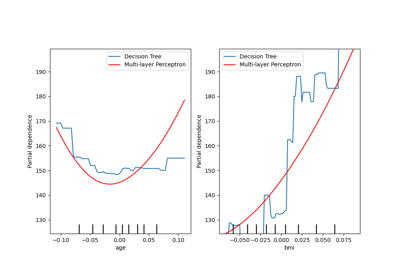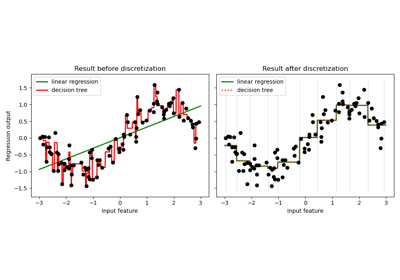# sklearn.tree.DecisionTreeRegressor¶

class sklearn.tree.DecisionTreeRegressor(*, criterion='squared_error', splitter='best', max_depth=None, min_samples_split=2, min_samples_leaf=1, min_weight_fraction_leaf=0.0, max_features=None, random_state=None, max_leaf_nodes=None, min_impurity_decrease=0.0, ccp_alpha=0.0)[source]

A decision tree regressor.

Read more in the User Guide.

Parameters:
criterion{“squared_error”, “friedman_mse”, “absolute_error”, “poisson”}, default=”squared_error”

The function to measure the quality of a split. Supported criteria are “squared_error” for the mean squared error, which is equal to variance reduction as feature selection criterion and minimizes the L2 loss using the mean of each terminal node, “friedman_mse”, which uses mean squared error with Friedman’s improvement score for potential splits, “absolute_error” for the mean absolute error, which minimizes the L1 loss using the median of each terminal node, and “poisson” which uses reduction in Poisson deviance to find splits.

New in version 0.18: Mean Absolute Error (MAE) criterion.

New in version 0.24: Poisson deviance criterion.

Deprecated since version 1.0: Criterion “mse” was deprecated in v1.0 and will be removed in version 1.2. Use criterion="squared_error" which is equivalent.

Deprecated since version 1.0: Criterion “mae” was deprecated in v1.0 and will be removed in version 1.2. Use criterion="absolute_error" which is equivalent.

splitter{“best”, “random”}, default=”best”

The strategy used to choose the split at each node. Supported strategies are “best” to choose the best split and “random” to choose the best random split.

max_depthint, default=None

The maximum depth of the tree. If None, then nodes are expanded until all leaves are pure or until all leaves contain less than min_samples_split samples.

min_samples_splitint or float, default=2

The minimum number of samples required to split an internal node:

• If int, then consider min_samples_split as the minimum number.

• If float, then min_samples_split is a fraction and ceil(min_samples_split * n_samples) are the minimum number of samples for each split.

Changed in version 0.18: Added float values for fractions.

min_samples_leafint or float, default=1

The minimum number of samples required to be at a leaf node. A split point at any depth will only be considered if it leaves at least min_samples_leaf training samples in each of the left and right branches. This may have the effect of smoothing the model, especially in regression.

• If int, then consider min_samples_leaf as the minimum number.

• If float, then min_samples_leaf is a fraction and ceil(min_samples_leaf * n_samples) are the minimum number of samples for each node.

Changed in version 0.18: Added float values for fractions.

min_weight_fraction_leaffloat, default=0.0

The minimum weighted fraction of the sum total of weights (of all the input samples) required to be at a leaf node. Samples have equal weight when sample_weight is not provided.

max_featuresint, float or {“auto”, “sqrt”, “log2”}, default=None

The number of features to consider when looking for the best split:

• If int, then consider max_features features at each split.

• If float, then max_features is a fraction and max(1, int(max_features * n_features_in_)) features are considered at each split.

• If “auto”, then max_features=n_features.

• If “sqrt”, then max_features=sqrt(n_features).

• If “log2”, then max_features=log2(n_features).

• If None, then max_features=n_features.

Deprecated since version 1.1: The "auto" option was deprecated in 1.1 and will be removed in 1.3.

Note: the search for a split does not stop until at least one valid partition of the node samples is found, even if it requires to effectively inspect more than max_features features.

random_stateint, RandomState instance or None, default=None

Controls the randomness of the estimator. The features are always randomly permuted at each split, even if splitter is set to "best". When max_features < n_features, the algorithm will select max_features at random at each split before finding the best split among them. But the best found split may vary across different runs, even if max_features=n_features. That is the case, if the improvement of the criterion is identical for several splits and one split has to be selected at random. To obtain a deterministic behaviour during fitting, random_state has to be fixed to an integer. See Glossary for details.

max_leaf_nodesint, default=None

Grow a tree with max_leaf_nodes in best-first fashion. Best nodes are defined as relative reduction in impurity. If None then unlimited number of leaf nodes.

min_impurity_decreasefloat, default=0.0

A node will be split if this split induces a decrease of the impurity greater than or equal to this value.

The weighted impurity decrease equation is the following:

N_t / N * (impurity - N_t_R / N_t * right_impurity
- N_t_L / N_t * left_impurity)


where N is the total number of samples, N_t is the number of samples at the current node, N_t_L is the number of samples in the left child, and N_t_R is the number of samples in the right child.

N, N_t, N_t_R and N_t_L all refer to the weighted sum, if sample_weight is passed.

New in version 0.19.

ccp_alphanon-negative float, default=0.0

Complexity parameter used for Minimal Cost-Complexity Pruning. The subtree with the largest cost complexity that is smaller than ccp_alpha will be chosen. By default, no pruning is performed. See Minimal Cost-Complexity Pruning for details.

New in version 0.22.

Attributes:
feature_importances_ndarray of shape (n_features,)

Return the feature importances.

max_features_int

The inferred value of max_features.

n_features_int

DEPRECATED: The attribute n_features_ is deprecated in 1.0 and will be removed in 1.2.

n_features_in_int

Number of features seen during fit.

New in version 0.24.

feature_names_in_ndarray of shape (n_features_in_,)

Names of features seen during fit. Defined only when X has feature names that are all strings.

New in version 1.0.

n_outputs_int

The number of outputs when fit is performed.

tree_Tree instance

The underlying Tree object. Please refer to help(sklearn.tree._tree.Tree) for attributes of Tree object and Understanding the decision tree structure for basic usage of these attributes.

DecisionTreeClassifier

A decision tree classifier.

Notes

The default values for the parameters controlling the size of the trees (e.g. max_depth, min_samples_leaf, etc.) lead to fully grown and unpruned trees which can potentially be very large on some data sets. To reduce memory consumption, the complexity and size of the trees should be controlled by setting those parameter values.

References



L. Breiman, J. Friedman, R. Olshen, and C. Stone, “Classification and Regression Trees”, Wadsworth, Belmont, CA, 1984.



T. Hastie, R. Tibshirani and J. Friedman. “Elements of Statistical Learning”, Springer, 2009.



L. Breiman, and A. Cutler, “Random Forests”, https://www.stat.berkeley.edu/~breiman/RandomForests/cc_home.htm

Examples

>>> from sklearn.datasets import load_diabetes
>>> from sklearn.model_selection import cross_val_score
>>> from sklearn.tree import DecisionTreeRegressor
>>> regressor = DecisionTreeRegressor(random_state=0)
>>> cross_val_score(regressor, X, y, cv=10)
...
...
array([-0.39..., -0.46...,  0.02...,  0.06..., -0.50...,
0.16...,  0.11..., -0.73..., -0.30..., -0.00...])


Methods

 apply(X[, check_input]) Return the index of the leaf that each sample is predicted as. cost_complexity_pruning_path(X, y[, ...]) Compute the pruning path during Minimal Cost-Complexity Pruning. decision_path(X[, check_input]) Return the decision path in the tree. fit(X, y[, sample_weight, check_input]) Build a decision tree regressor from the training set (X, y). Return the depth of the decision tree. Return the number of leaves of the decision tree. get_params([deep]) Get parameters for this estimator. predict(X[, check_input]) Predict class or regression value for X. score(X, y[, sample_weight]) Return the coefficient of determination of the prediction. set_params(**params) Set the parameters of this estimator.
apply(X, check_input=True)[source]

Return the index of the leaf that each sample is predicted as.

New in version 0.17.

Parameters:
X{array-like, sparse matrix} of shape (n_samples, n_features)

The input samples. Internally, it will be converted to dtype=np.float32 and if a sparse matrix is provided to a sparse csr_matrix.

check_inputbool, default=True

Allow to bypass several input checking. Don’t use this parameter unless you know what you do.

Returns:
X_leavesarray-like of shape (n_samples,)

For each datapoint x in X, return the index of the leaf x ends up in. Leaves are numbered within [0; self.tree_.node_count), possibly with gaps in the numbering.

cost_complexity_pruning_path(X, y, sample_weight=None)[source]

Compute the pruning path during Minimal Cost-Complexity Pruning.

See Minimal Cost-Complexity Pruning for details on the pruning process.

Parameters:
X{array-like, sparse matrix} of shape (n_samples, n_features)

The training input samples. Internally, it will be converted to dtype=np.float32 and if a sparse matrix is provided to a sparse csc_matrix.

yarray-like of shape (n_samples,) or (n_samples, n_outputs)

The target values (class labels) as integers or strings.

sample_weightarray-like of shape (n_samples,), default=None

Sample weights. If None, then samples are equally weighted. Splits that would create child nodes with net zero or negative weight are ignored while searching for a split in each node. Splits are also ignored if they would result in any single class carrying a negative weight in either child node.

Returns:
ccp_pathBunch

Dictionary-like object, with the following attributes.

ccp_alphasndarray

Effective alphas of subtree during pruning.

impuritiesndarray

Sum of the impurities of the subtree leaves for the corresponding alpha value in ccp_alphas.

decision_path(X, check_input=True)[source]

Return the decision path in the tree.

New in version 0.18.

Parameters:
X{array-like, sparse matrix} of shape (n_samples, n_features)

The input samples. Internally, it will be converted to dtype=np.float32 and if a sparse matrix is provided to a sparse csr_matrix.

check_inputbool, default=True

Allow to bypass several input checking. Don’t use this parameter unless you know what you do.

Returns:
indicatorsparse matrix of shape (n_samples, n_nodes)

Return a node indicator CSR matrix where non zero elements indicates that the samples goes through the nodes.

property feature_importances_

Return the feature importances.

The importance of a feature is computed as the (normalized) total reduction of the criterion brought by that feature. It is also known as the Gini importance.

Warning: impurity-based feature importances can be misleading for high cardinality features (many unique values). See sklearn.inspection.permutation_importance as an alternative.

Returns:
feature_importances_ndarray of shape (n_features,)

Normalized total reduction of criteria by feature (Gini importance).

fit(X, y, sample_weight=None, check_input=True)[source]

Build a decision tree regressor from the training set (X, y).

Parameters:
X{array-like, sparse matrix} of shape (n_samples, n_features)

The training input samples. Internally, it will be converted to dtype=np.float32 and if a sparse matrix is provided to a sparse csc_matrix.

yarray-like of shape (n_samples,) or (n_samples, n_outputs)

The target values (real numbers). Use dtype=np.float64 and order='C' for maximum efficiency.

sample_weightarray-like of shape (n_samples,), default=None

Sample weights. If None, then samples are equally weighted. Splits that would create child nodes with net zero or negative weight are ignored while searching for a split in each node.

check_inputbool, default=True

Allow to bypass several input checking. Don’t use this parameter unless you know what you do.

Returns:
selfDecisionTreeRegressor

Fitted estimator.

get_depth()[source]

Return the depth of the decision tree.

The depth of a tree is the maximum distance between the root and any leaf.

Returns:
self.tree_.max_depthint

The maximum depth of the tree.

get_n_leaves()[source]

Return the number of leaves of the decision tree.

Returns:
self.tree_.n_leavesint

Number of leaves.

get_params(deep=True)[source]

Get parameters for this estimator.

Parameters:
deepbool, default=True

If True, will return the parameters for this estimator and contained subobjects that are estimators.

Returns:
paramsdict

Parameter names mapped to their values.

property n_features_

DEPRECATED: The attribute n_features_ is deprecated in 1.0 and will be removed in 1.2. Use n_features_in_ instead.

predict(X, check_input=True)[source]

Predict class or regression value for X.

For a classification model, the predicted class for each sample in X is returned. For a regression model, the predicted value based on X is returned.

Parameters:
X{array-like, sparse matrix} of shape (n_samples, n_features)

The input samples. Internally, it will be converted to dtype=np.float32 and if a sparse matrix is provided to a sparse csr_matrix.

check_inputbool, default=True

Allow to bypass several input checking. Don’t use this parameter unless you know what you do.

Returns:
yarray-like of shape (n_samples,) or (n_samples, n_outputs)

The predicted classes, or the predict values.

score(X, y, sample_weight=None)[source]

Return the coefficient of determination of the prediction.

The coefficient of determination $$R^2$$ is defined as $$(1 - \frac{u}{v})$$, where $$u$$ is the residual sum of squares ((y_true - y_pred)** 2).sum() and $$v$$ is the total sum of squares ((y_true - y_true.mean()) ** 2).sum(). The best possible score is 1.0 and it can be negative (because the model can be arbitrarily worse). A constant model that always predicts the expected value of y, disregarding the input features, would get a $$R^2$$ score of 0.0.

Parameters:
Xarray-like of shape (n_samples, n_features)

Test samples. For some estimators this may be a precomputed kernel matrix or a list of generic objects instead with shape (n_samples, n_samples_fitted), where n_samples_fitted is the number of samples used in the fitting for the estimator.

yarray-like of shape (n_samples,) or (n_samples, n_outputs)

True values for X.

sample_weightarray-like of shape (n_samples,), default=None

Sample weights.

Returns:
scorefloat

$$R^2$$ of self.predict(X) wrt. y.

Notes

The $$R^2$$ score used when calling score on a regressor uses multioutput='uniform_average' from version 0.23 to keep consistent with default value of r2_score. This influences the score method of all the multioutput regressors (except for MultiOutputRegressor).

set_params(**params)[source]

Set the parameters of this estimator.

The method works on simple estimators as well as on nested objects (such as Pipeline). The latter have parameters of the form <component>__<parameter> so that it’s possible to update each component of a nested object.

Parameters:
**paramsdict

Estimator parameters.

Returns:
selfestimator instance

Estimator instance.

## Examples using sklearn.tree.DecisionTreeRegressor¶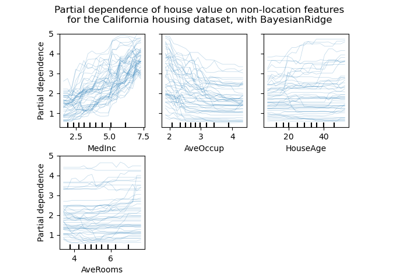Release Highlights for scikit-learn 0.24

Release Highlights for scikit-learn 0.24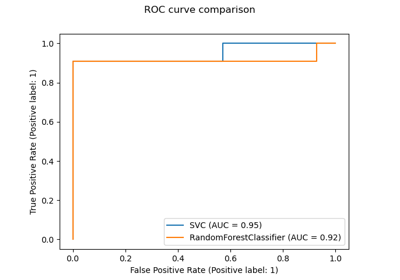Release Highlights for scikit-learn 0.22

Release Highlights for scikit-learn 0.22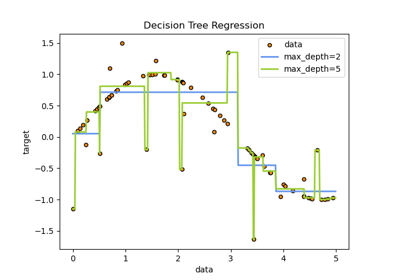Decision Tree Regression

Decision Tree Regression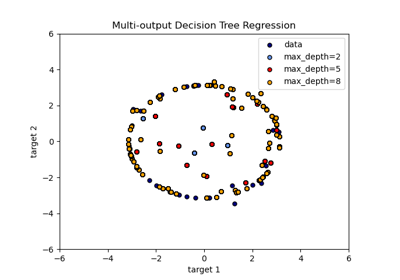Multi-output Decision Tree Regression

Multi-output Decision Tree Regression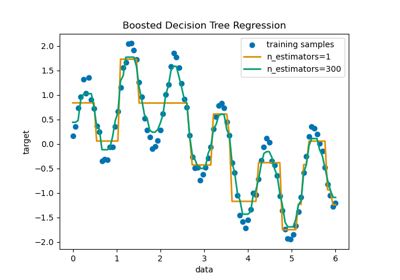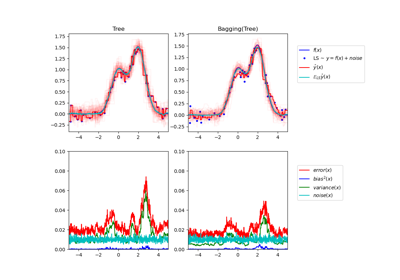Single estimator versus bagging: bias-variance decomposition

Single estimator versus bagging: bias-variance decomposition